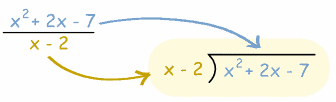Dividing Polynomials
4 years ago
derrickalmeida
Save
Edit
Host a game
Live GameLive
Homework
Solo Practice
Practice17 QuestionsShow answers
• Question 1
300 seconds
Q. Divide:
(6x + 9) / (3x)
2 + 9/3x
2 + 9
2 + 9/3
2
• Question 2
300 seconds
Q. Divide:
(24x + 4) / (6x+1)
4
4 + 4/6x+1
4x + 0
4x
• Question 3
300 seconds
Q. Divide:
(9x2 + 6) / (3x)
3x + 6/3x
3x + 2
3 + 6/3x
3x + 6
• Question 4
300 seconds
Q. Divide:

(4x2 - 24x + 35) / (2x - 5)
2x - 7
2x - 12
2x + 12
2x + 7
• Question 5
180 seconds
Q. Find the product:
(y - 3)(y + 7)
y2 - 21
y2 + 10y - 21
y2 + 4y - 21
2y - 10
• Question 6
180 seconds
Q.  Multiply:
(r + 7)(r − 7)
r 2 − 49
r 2 + 14
r 2 − 7r + 49
• Question 7
180 seconds
Q. Simplify
(p − 8)2

Hint: (p − 8)2 = (p − 8)(p − 8)
p 2 − 16p − 64
p 2 + 16p + 64
p 2 − 16p − 8
p 2 − 16p + 64
• Question 8
120 seconds
Q. What type of polynomial is
4x2 - 21x + 5
Monomial
Binomial
Trinomial
• Question 9
120 seconds
Q. What type of polynomial is
4x+ 2
Monomial
Binomial
Trinomial
• Question 10
120 seconds
Q. What type of polynomial is
2x2
Monomial
Binomial
Trinomial
• Question 11
120 seconds
Q. What is the degree of the following polynomial?
2x3
First Degree
Second Degree
Third Degree
Fourth Degree
• Question 12
120 seconds
Q. What is the degree of the following polynomial?
3x4 + 4x + 1
First Degree
Second Degree
Third Degree
Fourth Degree
• Question 13
300 seconds
Q. which binomial product would produce this as an answer?
x2 - 100
(x - 10)(x +10)
(x - 2)(x + 50)
(x - 4)(x + 25)
(x - 1)(x + 100)
• Question 14
120 secondsQ. Remember to combine like terms
CLICK THIS TO HIDE TEXT
A
B
C
D
• Question 15
120 secondsQ. Remember to combine like terms
CLICK THIS TO HIDE TEXT
A
B
C
D
• Question 16
120 secondsQ. Remember to combine like terms
CLICK THIS TO HIDE TEXT
A
B
C
D
• Question 17
120 secondsQ. Remember to combine like terms
CLICK THIS TO HIDE TEXT